# Proportion

Go back to  'Commercial Math'

 1 Introduction to Proportion 2 Basics of Proportions 3 Tips and Tricks 4 Properties of Proportion 5 Important Notes on Proportion 6 Solved Examples on Proportion 7 Practice Questions on Proportion 8 Maths Olympiad Sample Papers 9 Frequently Asked Questions (FAQs) 10 Download FREE Worksheets on Proportion

We at Cuemath believe that Math is a life skill. Our Math Experts focus on the “Why” behind the “What.” Students can explore from a huge range of interactive worksheets, visuals, simulations, practice tests, and more to understand a concept in depth.

Book a FREE trial class today! and experience Cuemath's LIVE Online Class with your child.

## Introduction to Proportion

### Proportion Definition

Proportion is a mathematical comparison between two numbers.

Let's say, your friend has invited you to a birthday party.

Your friend has also invited other children from your school and tuition.

You are excited because you are good friends with some children from school and some from the tuition, and you can't wait to play with all of them at the party.

You want to know how many of the attendees are your friends from school and how many are your friends from your tuition.

We can do this comparison in the form of proportions.

By counting the numbers under each group, you will get an idea of how many of those attendees are your friends from each group.

You just found out that 5 out of 12 children are from your tuition and the rest are from your school.

This is how we use proportions.

Now, lets understand this concept in detail.

So, what is a proportion?

According to the proportion definition, we say that two ratios are equivalent, then it is called a proportion.

### Proportion Formula

We write it as a : b : : c : d or a : b = c : d

We read it as “a is to b as c is to d”.

A proportion means two ratios which have been set equal to each other.

A proportion formula is an equation that can be solved to get the comparison values.

To solve proportion problems, we use the concept that, proportion is two ratios that are equal to each other, we mean this in the sense of two fractions being equal to each other.

For instance,

\begin{align}5 : 10 = \frac{5}{10} = \frac{1}{2} = 1 : 2\end{align}

## Basics of Proportions

There are two types of proportions.

• Direct Proportion
• Indirect Proportion

### Direct Proportion

There are many situations in our daily lives that involve using direct proportion.

Example:

Jane ran 100 meters in 15 seconds.

How long will she take to run 2 meters?

Will she take longer than 15 seconds or lesser than 15 seconds?

Let's see how to solve this example using proportions.

In this example, meters (distance) is in proportion to seconds (time taken).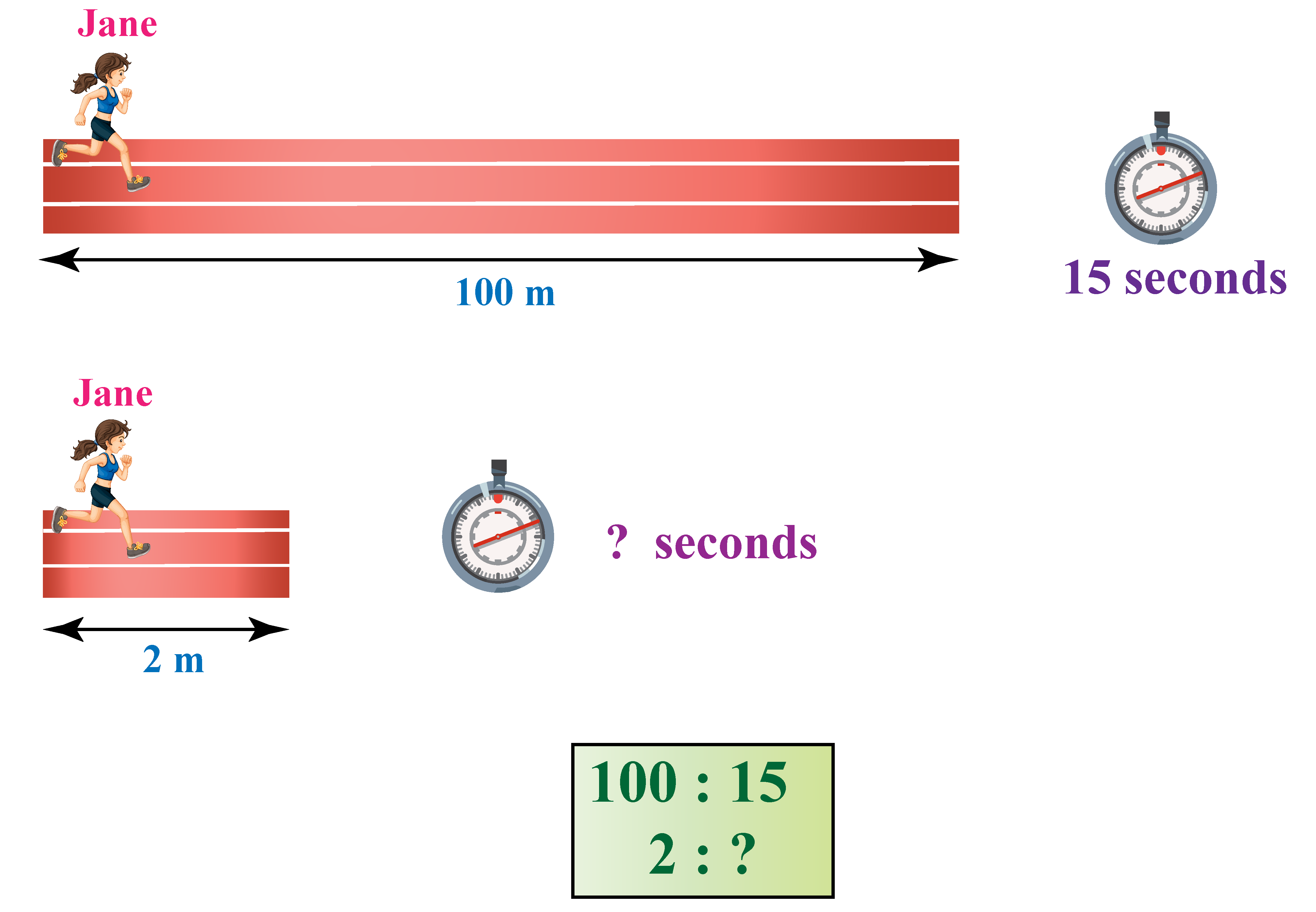Time taken by Jane to run 100 meters = 15 seconds

Let's assume that the time taken by her to run 2 meters = $$x$$  seconds

We can say that:

\begin{align} \frac{100}{15}&=\frac{2}{x} \\ x&=\frac{2\times 15}{100}\end{align}

Hence,

\begin{align} x=0.3\end{align}

Thus, she took 0.3 seconds to cover 2 meters.

Here, the distance covered is directly proportional to the time taken.

In other words, as distance increases, the time taken to cover that distance increases and vice verse.

### Inverse Proportion

There are many situations in our daily lives that involve inverse proportion.

For example, the number of days required to build a wall is inversely proportional to the number of workers.

As the number of workers increases, the number of days required to build the wall decreases.

You can visualize this example using the simulation below.

The two quantities, the number of workers $$x$$ and the number of days $$y$$ required are related in such a way that when one quantity increases, the other decreases.

In general, when two variables $$x$$  and $$y$$ are such that \begin{align}\frac{x}{y}=k\end{align}

where $$k$$ is a non-zero constant, we say that $$y$$ is inversely proportional to $$x$$

In notation, inverse proportion is written as:

 \begin{align}y\propto\frac{1}{x}\end{align}Tips and Tricks
1. Proportions can be direct or indirect.
2. A trick is to always think of the situation from real life and determine whether we are dealing with direct or inverse proportions.

### Comparison between Direct Proportion and Indirect Proportion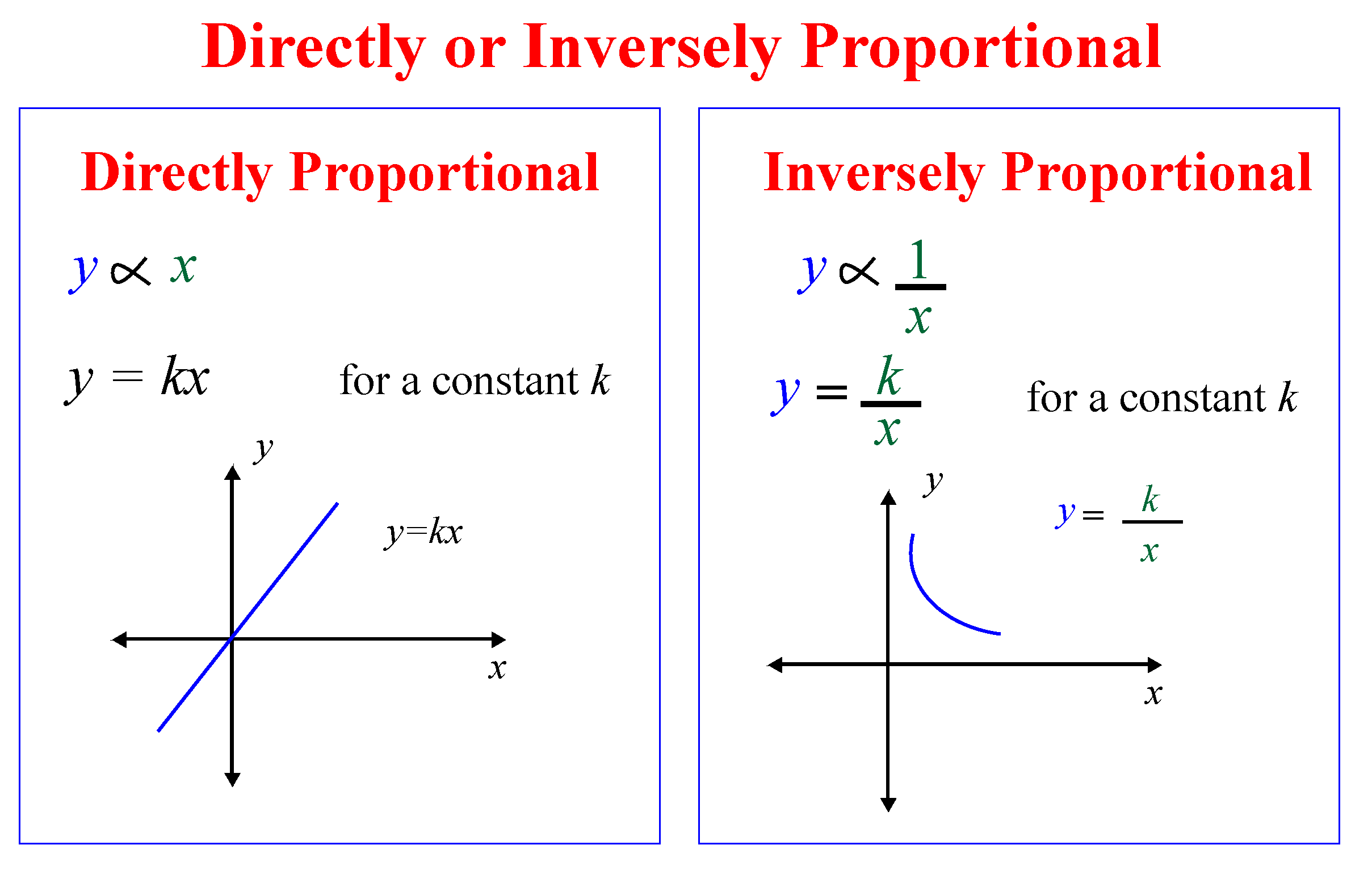In the figure above,

Directly Proportional: Here $$y$$ is directly proportional to $$x$$. As the $$x$$ value increases $$y$$ graph increases also.

Indirectly Proportional: Here $$y$$ is indirectly proportional to $$x$$. As the $$x$$ value increases $$y$$ graph decreases.

## Properties of Proportion

In this section, you will learn about the important properties of proportion which will help you in proportion calculations.

### 1. Reciprocal Property

If two ratios are equal, then their reciprocals must also be equal as long as they exist.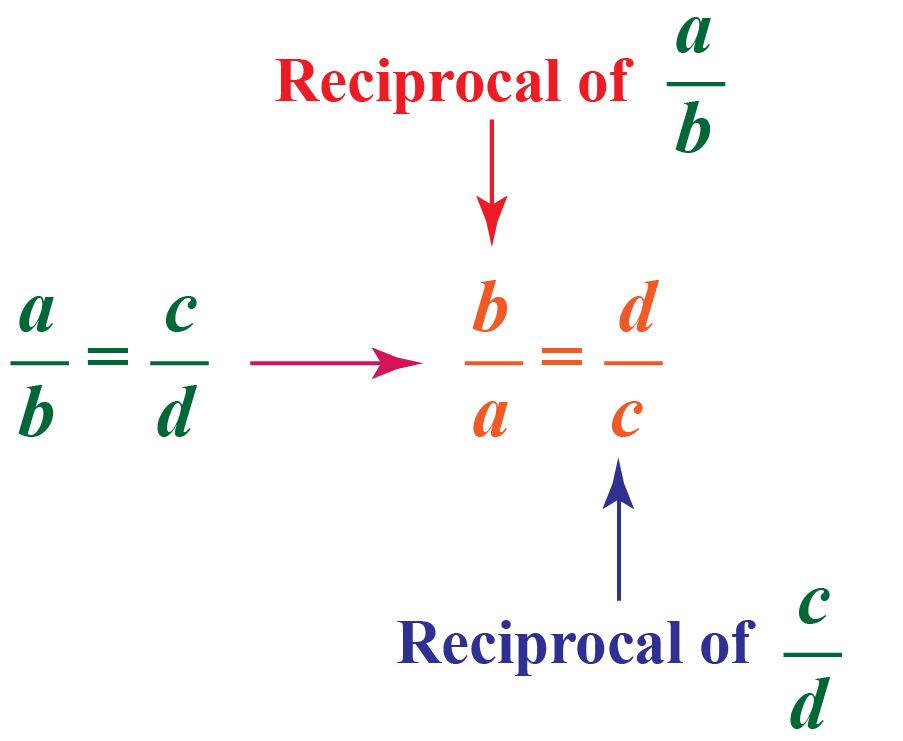### 2. Cross Product Property

The product of the extremes is equal to the product of the means.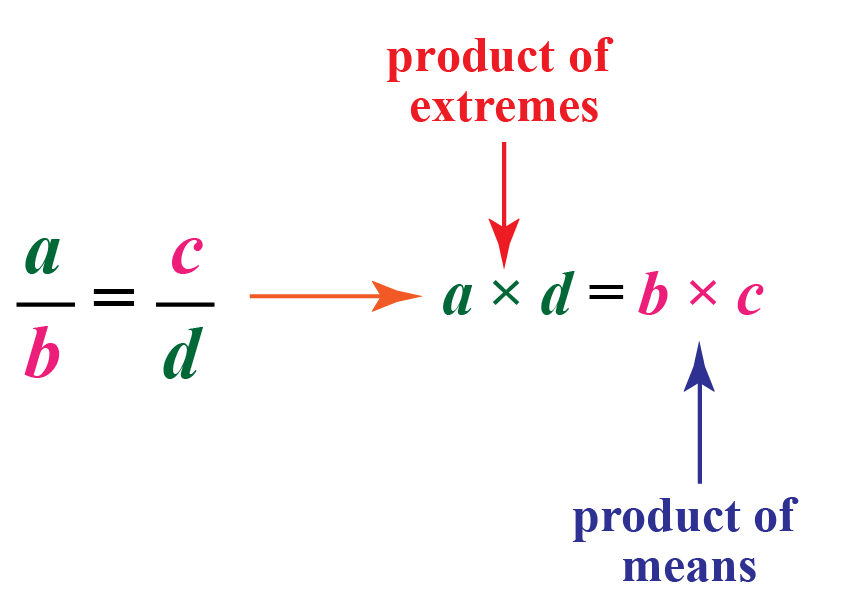### 3. Invertendo

If a : b = c : d, then b : a = d : c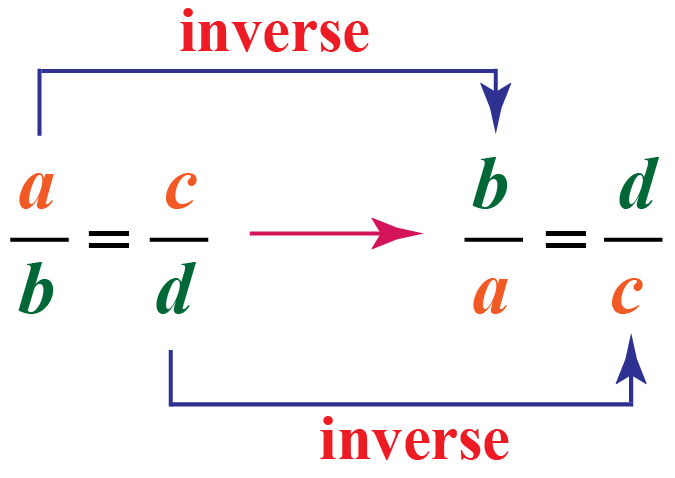### 4. Alternendo

If a : b = c : d, then a : c = b : d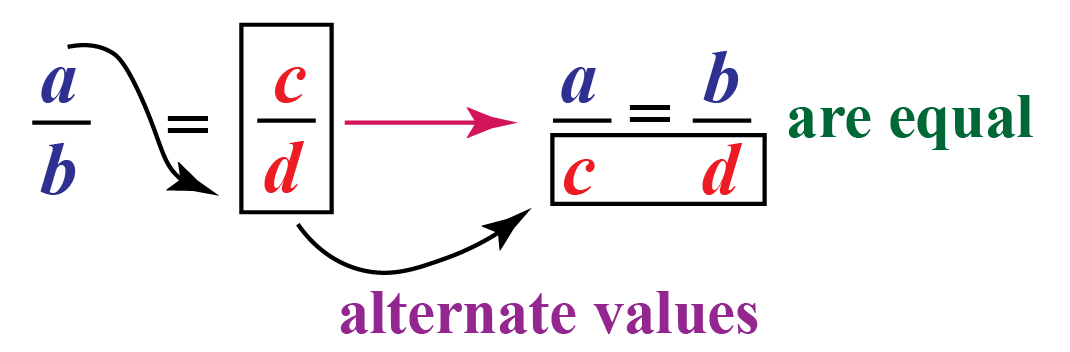### 5. Componendo

If a : b = c : d, then (a+b) : b  =  (c+d) : d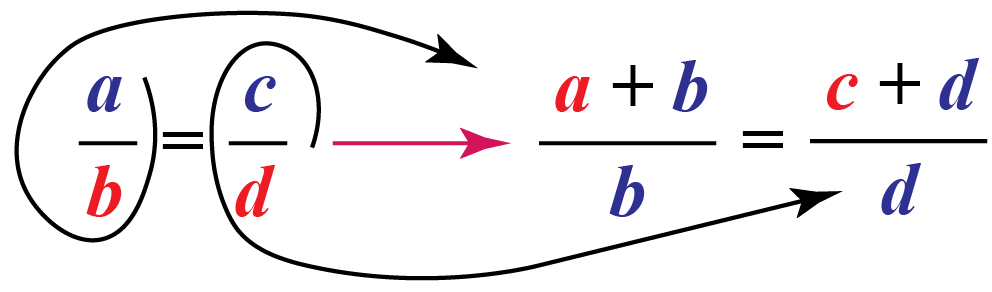### 6. Dividendo

If a : b = c : d, then (a-b) : b  =  (c-d) : d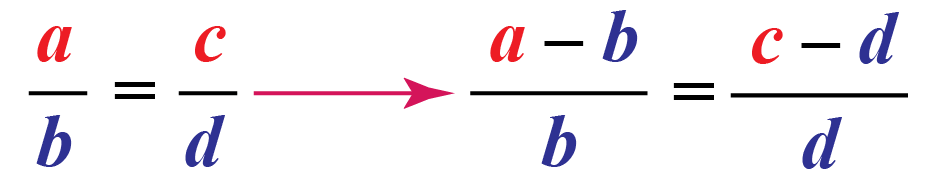### 7. Componendo and Dividendo

If a : b = c : d, then (a+b) : (a-b)  =  (c+d) : (c-d)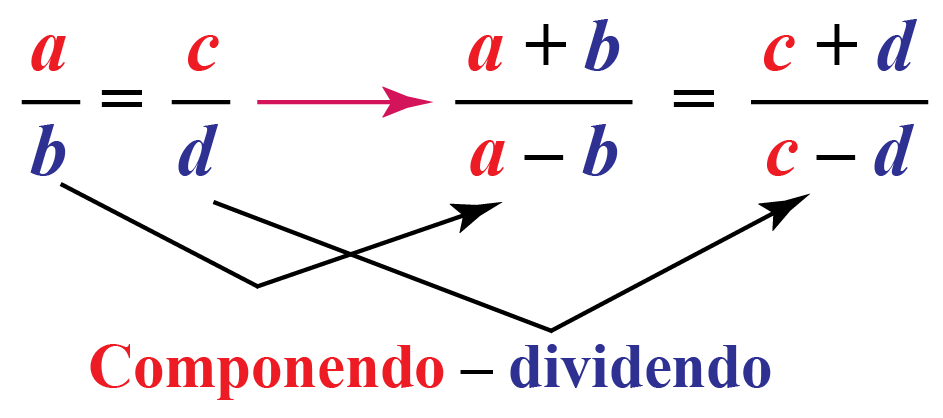The properties of proportion are summarized in the table below.

 Invertendo \begin{align}\frac{a}{b} = \frac{c}{d} \implies \frac{b}{a}=\frac{d}{c}\end{align} Alternendo \begin{align}\frac{a}{b} = \frac{c}{d} \implies \frac{a}{c}=\frac{b}{d}\end{align} Componendo \begin{align}\frac{a}{b} = \frac{c}{d} \implies \frac{a+b}{b}=\frac{c+d}{d}\end{align} Dividendo \begin{align}\frac{a}{b} = \frac{c}{d} \implies \frac{a-b}{b}=\frac{c-d}{d}\end{align} Componendo and Dividendo \begin{align}\frac{a}{b} = \frac{c}{d} \implies \frac{a+b}{a-b}=\frac{c+d}{c-d}\end{align}

Help your child score higher with Cuemath’s proprietary FREE Diagnostic Test. Get access to detailed reports, customized learning plans, and a FREE counseling session. Attempt the test now.

## Solved Examples on Proportions

 Example 1

My mother's recipe to make pancakes says, "to make 20 pancakes, you have to use 2 eggs."

How many eggs will I need to make 100 pancakes?

Will I need a dozen eggs or half a dozen?

Eggs Pancakes
Small amount 2 20
Large amount $$x$$ 100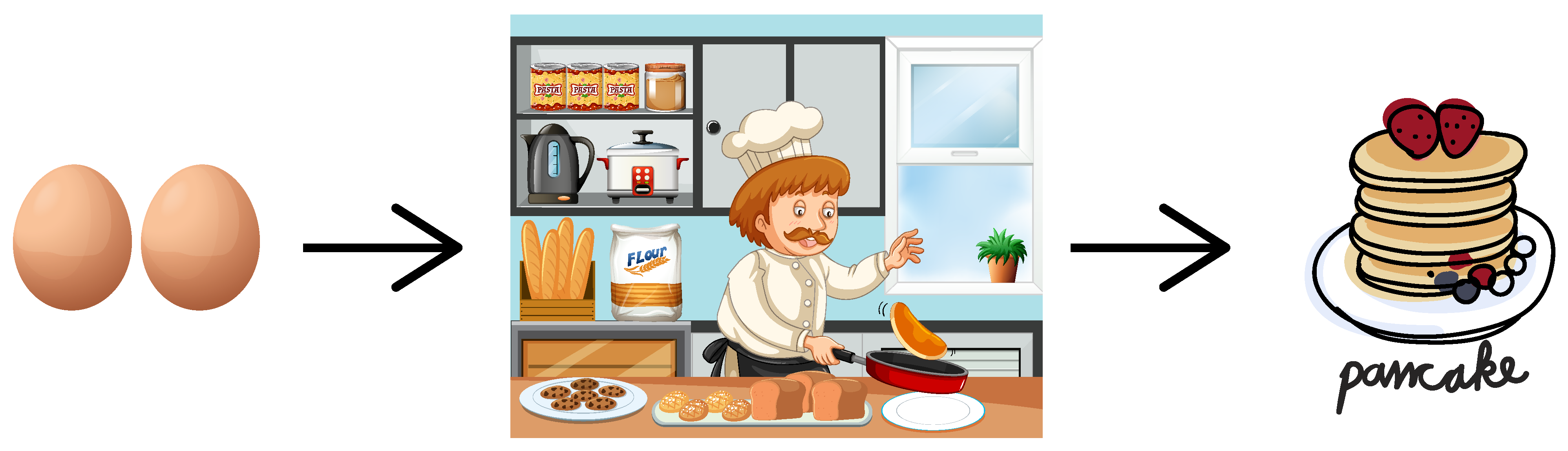Hint:

2 eggs make 20 pancakes

? eggs make 100 pancakes

Solution:

We know that:

$Eggs\propto \ pancakes$

If we write the unknown number in the numerator, then we can solve this as any other equation.

\begin{align}\frac{x}{100}=\frac{2}{20}\end{align}
Multiplying 100 on both sides:

\begin{align}100\times\frac{x}{100}&=100\times\frac{2}{20}\\ x&=\frac{200}{20} \\ x&=10\end{align}

 $$\therefore$$ We need 10 eggs to make 100 pancakes.
 Example 2

Jessy runs 4 km in 30 minutes.

At this rate, how far could she run in 45 minutes?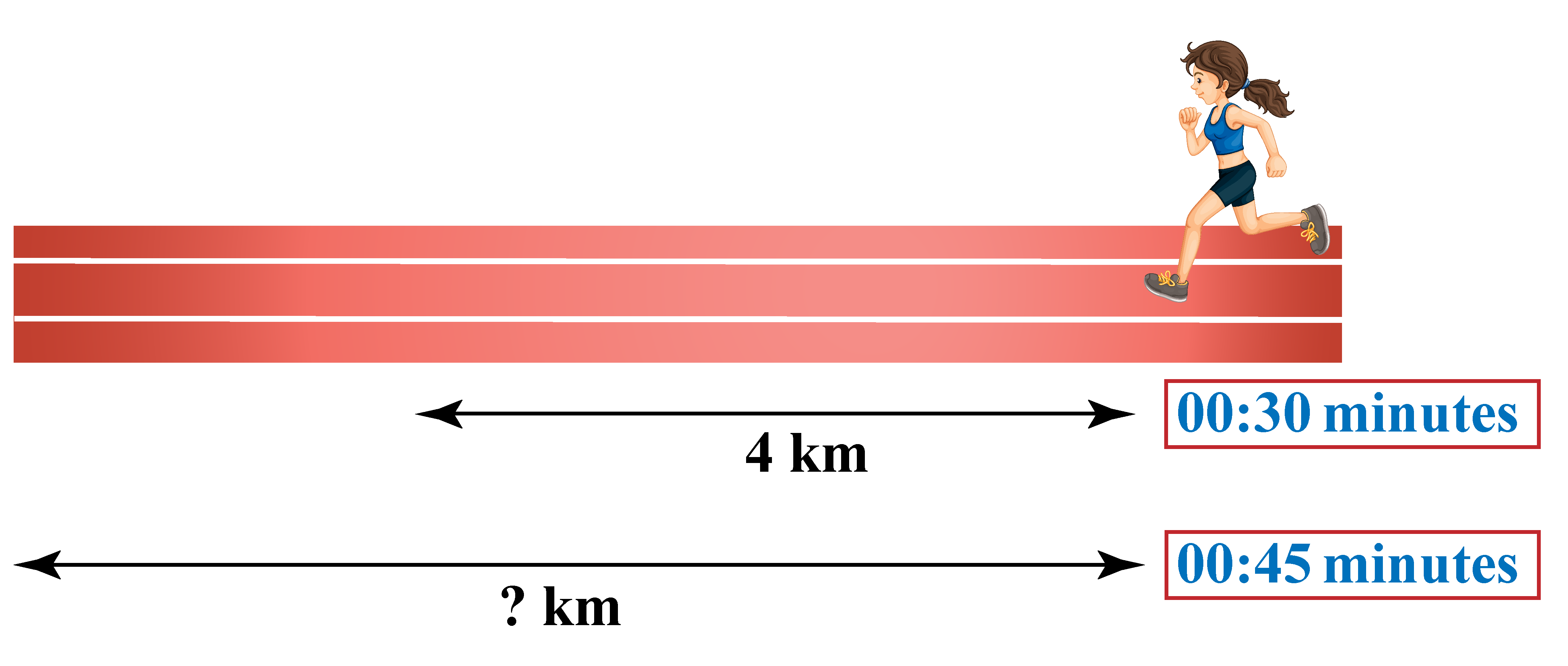Solution:

Let's assume the unknown quantity here is  $$x$$.

In this case, $$x$$ is the number of km Jessy can run in 45 minutes at the given rate.

It's given that running 4 km in 30 minutes is the same as running $$x$$ km in 45 minutes.

Hence, if we do the proportion math, we get :

\begin{align}\frac{4\:\text{km}}{30\:\text{min}} &= \frac{x\:\text{km}}{45\:\text{mins}} \\ \frac{4}{30} &= \frac{x}{45}\end{align}

Finding the cross products and setting them equal, we get:

\begin{align}30 \;x = 4\times 45 \end{align}

or

\begin{align}30\times x = 180\end{align}

Dividing both sides by 30, we get:

\begin{align}x =180\div 30 = 6\end{align}

 $$\therefore$$ Jessy can run 6 km in 45 minutes.
 Example 3

In an apartment, nine taps can fill a full tank in four hours.

How long do you think it will take if twelve taps of similar flowrate are used to fill the same tank?

Does it fill the tank faster or does it slow the process?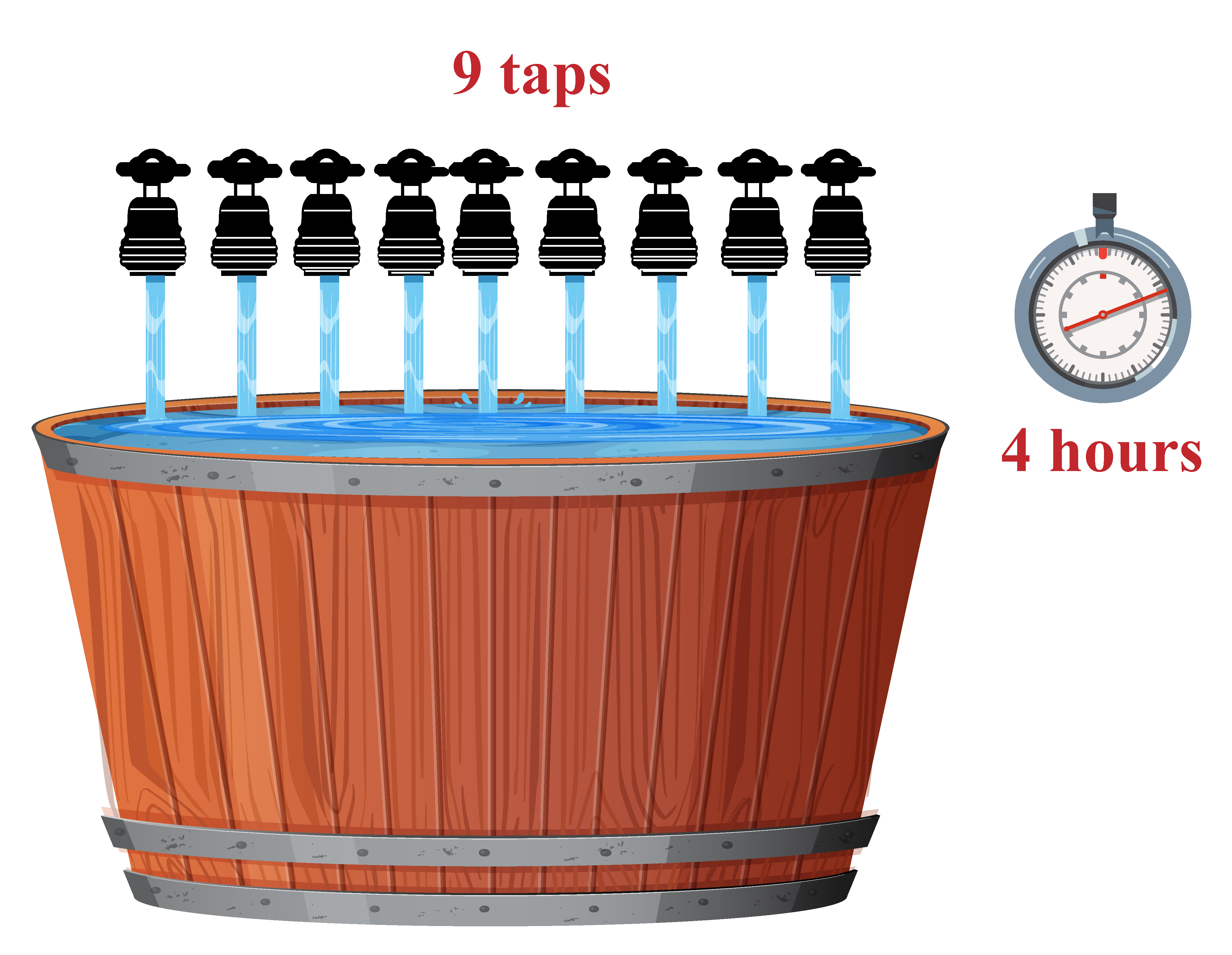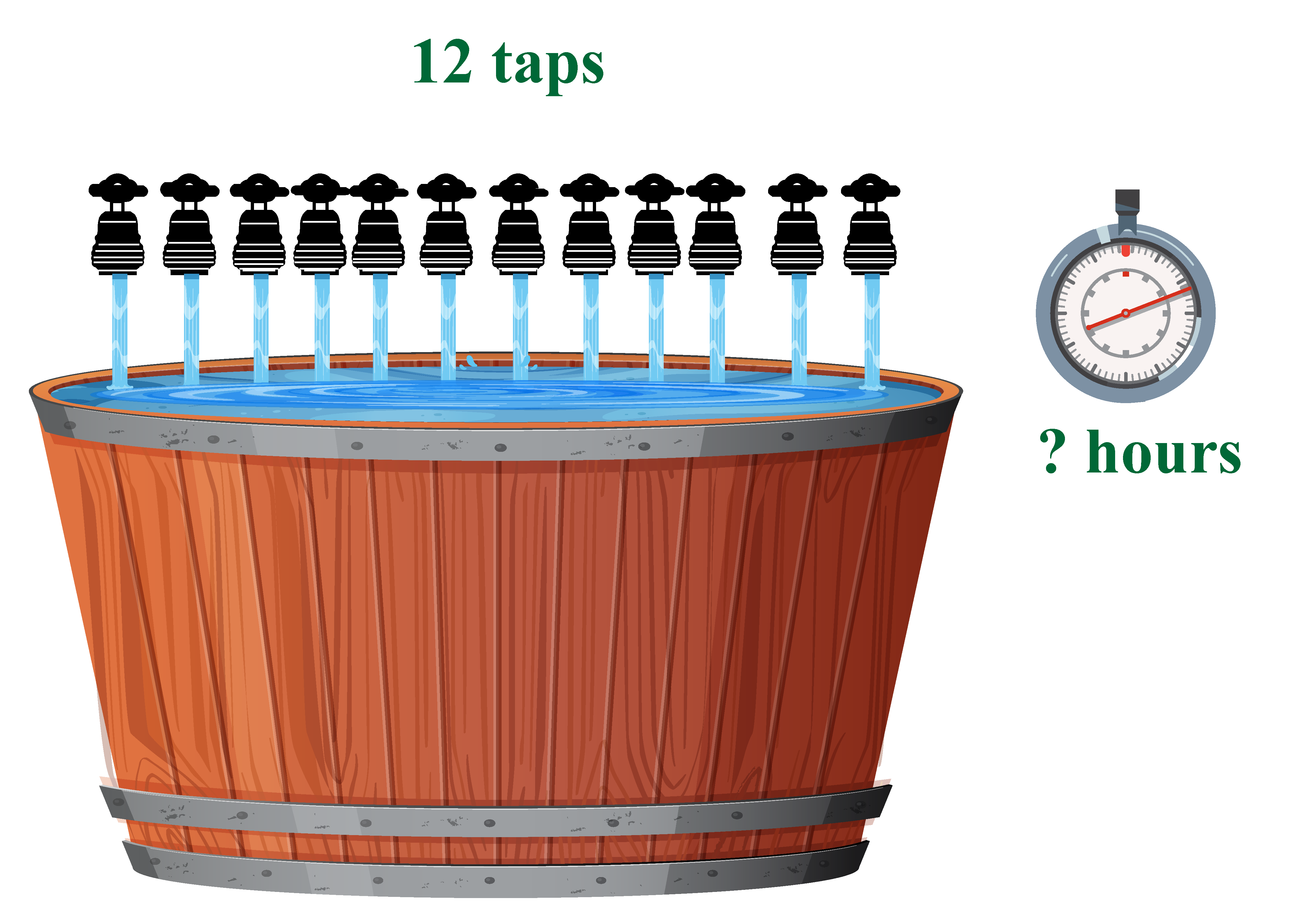Solution:

Given that,

It takes nine taps to fill the tank in 4 hours.

This is inverse proportion problem, so,

Let's assume that the time taken to fill the same tank using 12 taps is $$x$$.

\begin{align}\frac{9}{x} &= \frac{12}{4} \\ x&=3 \end{align}

Therefore, as the number of taps increases, inversely the time taken to fill the tank decreases.

So, 12 taps fill the tank much faster than 9 taps.

 $$\therefore$$ 12 taps will take 3 hours and fill the tank faster.
 Example 4

If a triangle ABC has a perimeter of 12 units and is similar to triangle RST with a scale factor of $$\frac{1}{3}$$, then find the perimeter of triangle RST.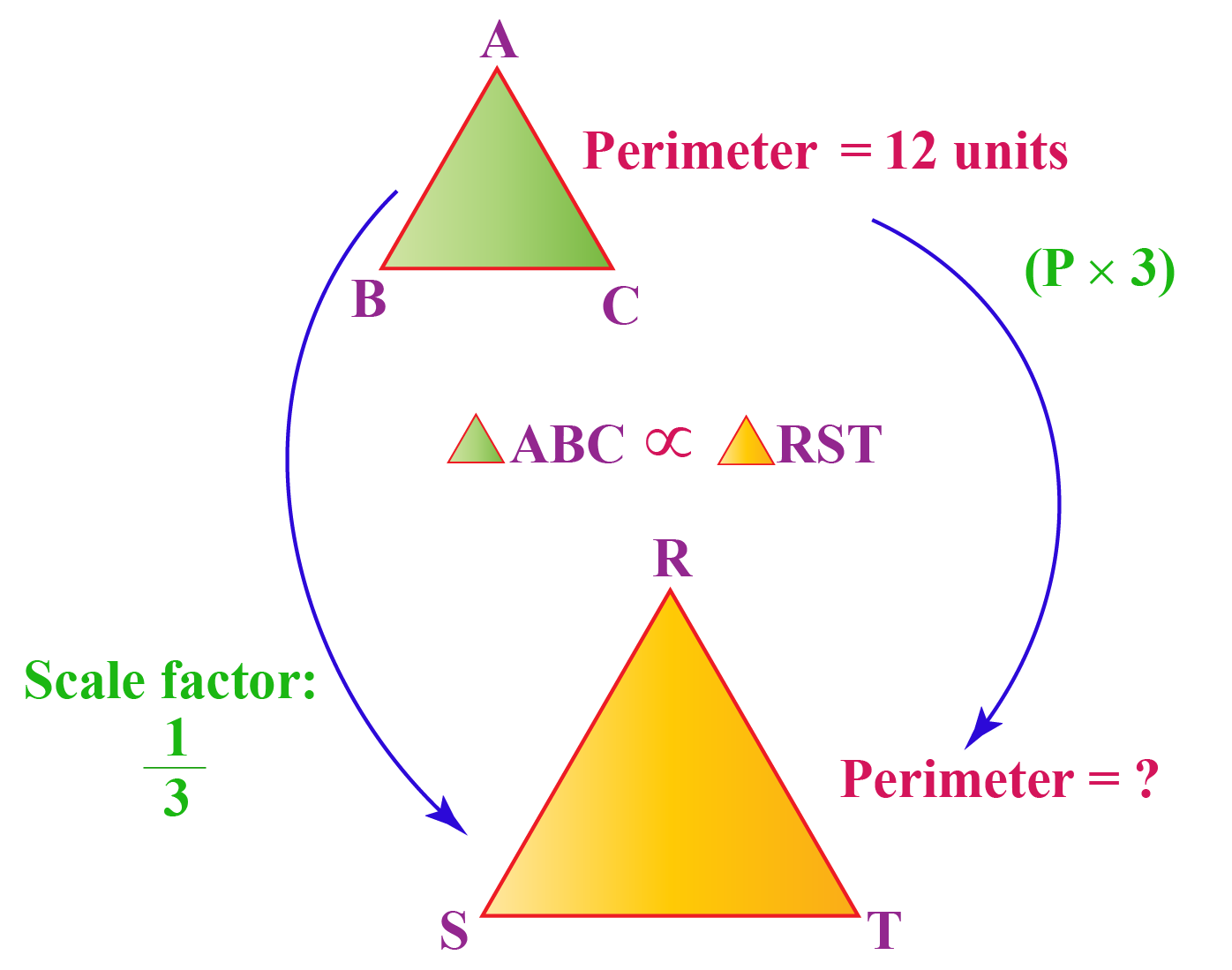Let $$x$$ represent the perimeter of triangle RST.

Scale factor implies that the perimeters are in proportion to this ratio.

The proportion will be as follows:

\begin{align} \frac{1}{3} = \frac{12}{x} \end{align}

Cross multiply and solve for $$x$$.

\begin{align}1\times x&= 3\times12 \\ x&= 36 \end{align}

 $$\therefore$$The perimeter of triangle RST is 36 units.
 Example 5

I want to make a nice fluffy cake on my mother's birthday.

To bake a perfect cake, I need to use sugar and flour in the proportion of 1:1

If I have 3 kg of sugar available to make a 6 kg cake, how many kilograms of flour is required?

The simulation below will show you the right mixing proportions for the cake.

Solution:

Let the quantity of flour required be $$x$$ kg.

We know that 3 kg of sugar is needed for a 6 kg cake.

We need to find the quantity of flour required for a 6 kg cake.

The proportion will be:

\begin{align} \frac{1}{1} &= \frac{x}{3} \\ x &=3 \end{align}

 $$\therefore$$ 3 kgs of flour is needed.

Want to understand the “Why” behind the “What”? Explore Proportion with our Math Experts in Cuemath’s LIVE, Personalised and Interactive Online Classes.

Make your kid a Math Expert, Book a FREE trial class today!

## Practice Questions on Proportions

Here are a few activities for you to practice.

IMO (International Maths Olympiad) is a competitive exam in Mathematics conducted annually for school students. It encourages children to develop their math solving skills from a competition perspective.

## 1. What are proportions?

Proportions are mathematical comparison between two numbers.

## 2. What is the proportion formula?

Proportion formula is indicated as a : b : : c : d or a : b = c : d

It reads as “a is to b as c is to d”.

## 3. What is proportion and ratio?

Ratio: The relative size of two quantities expressed as the quotient of one divided by the other; the ratio of a to b is written as a:b or a/b.

Proportion: An equality between two ratios.

## 4. What is direct proportion? Give an example.

When two variables $$x$$ and $$y$$ are such that the ratio remains a constant, we say that y is directly proportional to x.

For Example, Distance is directly proportional to time taken to travel. As the distance increases, the time taken to cover that distance also increases.

## 5. What are the 3 kinds of proportion?

• Direct proportion

• Inverse proportion

• Partitative proportion

Ratio, Proportion, and Variation
Ratio, Proportion, and Variation
Ratio and Variation